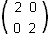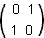Question: What do you mean by Matrix Transformation ? Answer: Transformations of the plane which map parallel lines onto parallel lines can be described by using 2 by 2 matrices. The matrix for an enlargement, centre (0, 0) with scale factor 2 isThe matrix for reflection in the line y = x is